# Graph - Adjacency Matrix Representation

### <<Previous Next >>

In this page, we will learn about Adjacency Matrix Representation of graph.

## Graph Representation

There are different ways to represent Graph - Adjacency Matrix Representation & Adjacency List Representation

### Adjacency Matrix Representation

One simple way is to use adjacency matrix representation which uses a two-dimensional array. Adjacency matrix is a square matrix with each entry indicating whether a pair of vertices are adjacent to each another.

• If there is an edge between two vertices vi and vj, then we set A[vi][vj]=1.
• Otherwise we set the entry in the array to 0.

For the above graph, For adjacency matrix, put 1 in the table as shown below if Vi and Vj have edge in the graph else put 0.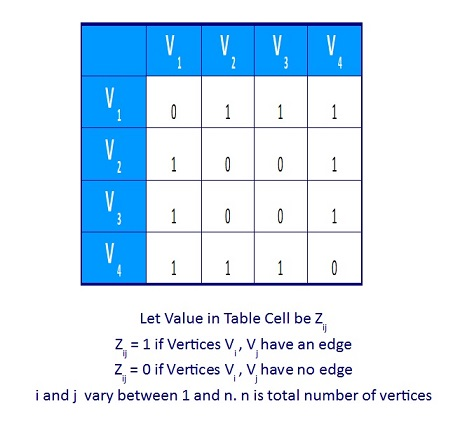Figure 4. Matrix Representation of Unweighted Graph

Suppose, if the edge is associated with weight, then we can set A[vi][vj] equal to the weight. The memory space required for this adjacency matrix is O(|V|2).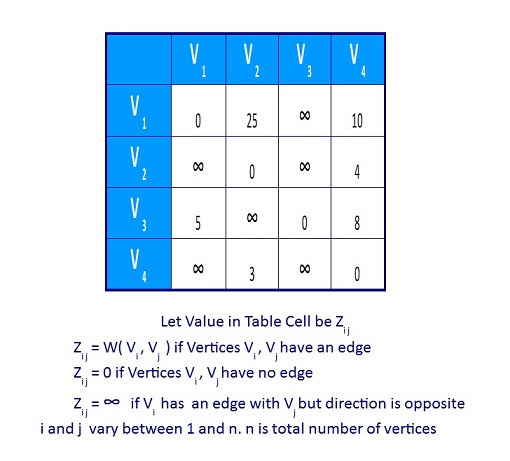Figure 5. Matrix Representation of Weighted Directed Graph

For adjacency matrix, put the weightage in the table if Vi and Vj have edge with weightage, else put some constant C. Here we take the constant as .The self ending edge weightage is 0. If the graph is dense, an adjacency matrix is suitable for graph representation.

# Graph - Adjacency Matrix Representation

### <<Previous Next >>

In this page, we will learn about Adjacency Matrix Representation of graph.

## Graph Representation

There are different ways to represent Graph - Adjacency Matrix Representation & Adjacency List Representation

### Adjacency Matrix Representation

One simple way is to use adjacency matrix representation which uses a two-dimensional array. Adjacency matrix is a square matrix with each entry indicating whether a pair of vertices are adjacent to each another.

• If there is an edge between two vertices vi and vj, then we set A[vi][vj]=1.
• Otherwise we set the entry in the array to 0.

For the above graph, For adjacency matrix, put 1 in the table as shown below if Vi and Vj have edge in the graph else put 0.Figure 4. Matrix Representation of Unweighted Graph

Suppose, if the edge is associated with weight, then we can set A[vi][vj] equal to the weight. The memory space required for this adjacency matrix is O(|V|2).Figure 5. Matrix Representation of Weighted Directed Graph

For adjacency matrix, put the weightage in the table if Vi and Vj have edge with weightage, else put some constant C. Here we take the constant as .The self ending edge weightage is 0. If the graph is dense, an adjacency matrix is suitable for graph representation.

### << Previous Next >>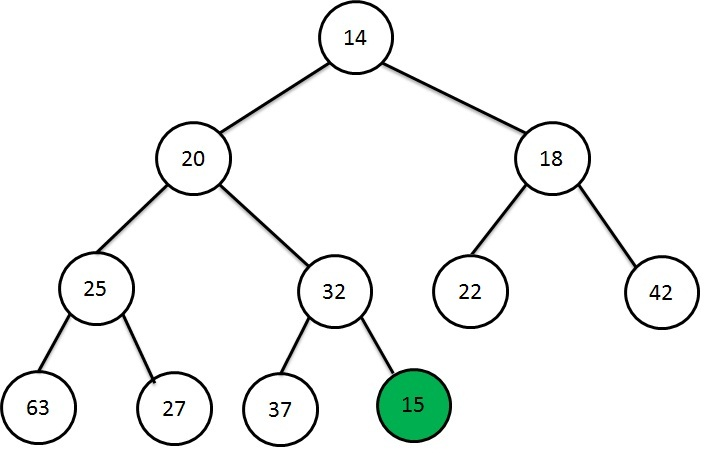Heap Data Structure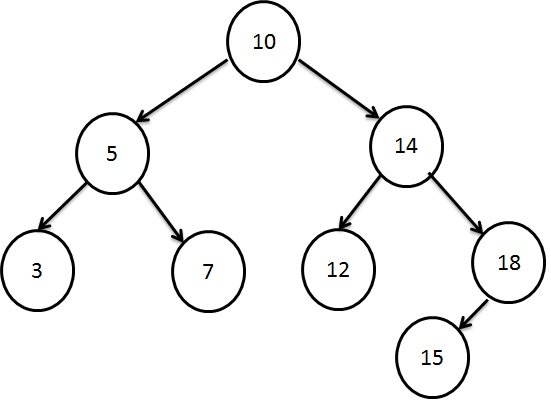Searching using Binary Search Tree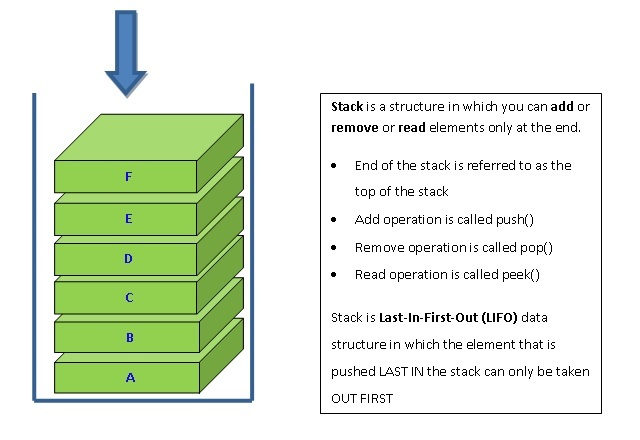Stacking the Data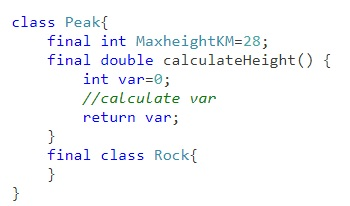Learn Java final keyword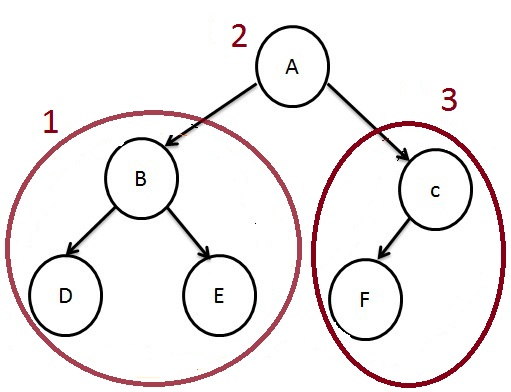How to traverse a Tree?